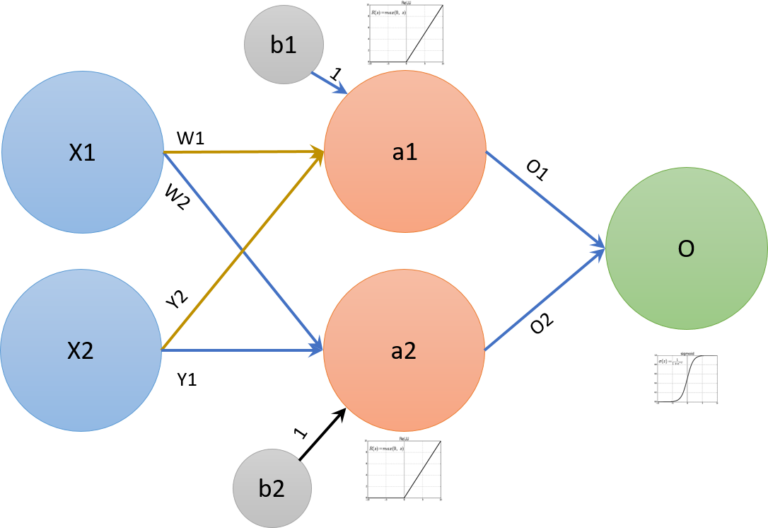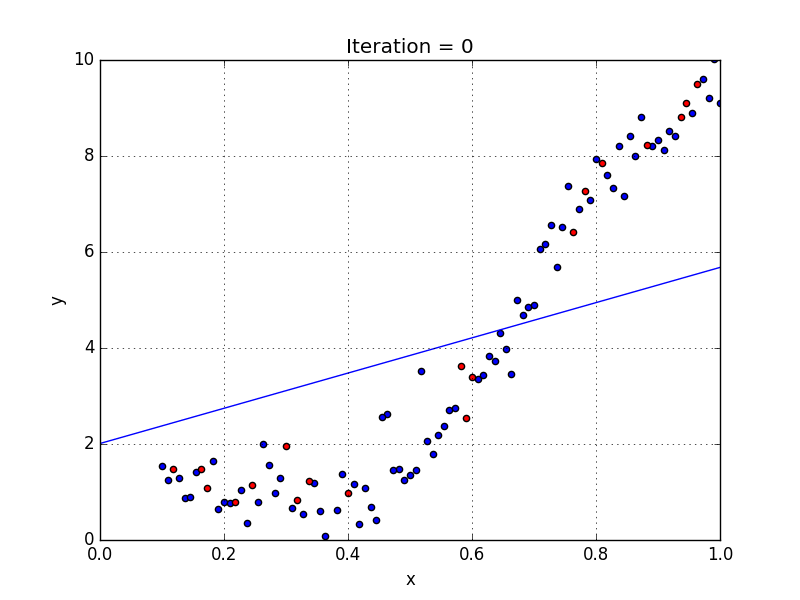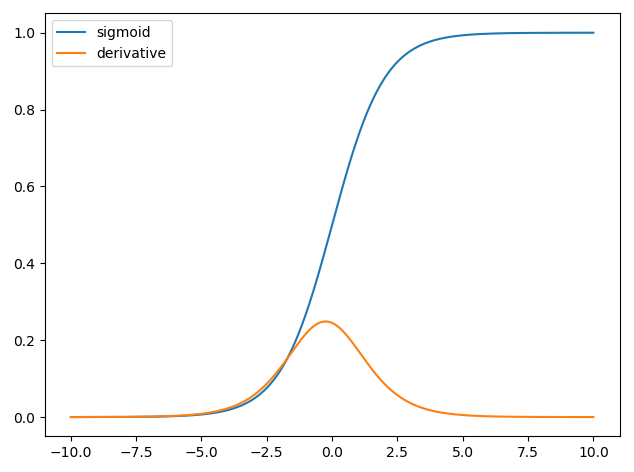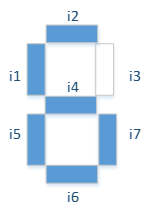## Let’s try to debunk the artificial neural networks and place them in the wider field of machine learning.

Nowadays machine learning is everywhere, and it has become the prevailing way to decline the concept of artificial intelligence.

It is one of the fields with the highest innovation rate ever, boosted by radical innovations leapfrogging each other relentlessly. However, the underlying concepts are unfortunately not easy to grasp. The idea here is to attempt to attempt to make the topic as much intuitive as possible.

Being non-trivial subjects, it is appropriate to make a premise: the purpose of this article is not to go into demonstrations or mathematical details. Each paragraph will be introduced by a brief description of the concept, before going down a little deeper.

In the Link section, there will be enough references for anyone who is interested in looking into the topic.

### What are neural networks? Beyond the definitions

Wikipedia gives this definition:

Artificial neural networks (ANNs) or connectionist systems are computing systems vaguely inspired by the biological neural networks that constitute animal brains.

This is certainly true, but unfortunately, it does not help much to understand what it is all about. The rest of the wiki, although very detailed, is quite difficult for those who do not already have some knowledge of the topic.

### Biological counterpart

Artificial neural networks (ANN) are algorithms used to solve complex problems that are not easy to code. We could say that they are the foundation of Machine Learning as we know it today.

The reason why they are called “neural networks” is because the nodes’ behavior recalls the behavior of biological neurons. A neuron receives signals in input from other neurons via synaptic connections, integrates them, and if the resulting activation exceeds a certain threshold it generates an Action Potential that propagates through its axon to one or more other neurons.#### ARTIFICIAL NEURAL NETWORKS IN PILLS

We can consider a neural network as a “black box”. It has inputs, intermediate layers in which “stuff happens”, and outputs that make up the final result.

The neural network is made of “units” called neurons, arranged in successive layers. Each neuron is typically connected to all the neurons of the next layer by weighted connections. A connection is nothing but a numerical value (the “weight”), which is multiplied by the value of the connected neuron.

Each neuron adds together the weighted values of all the neurons connected to itself and adds a bias value. An “activation function” is applied to this sum, which just transforms mathematically the value before passing it to the next layer. This way the input values are propagated through the network up to the output neurons. This is practically all that a neural network does.

The essence of everything is to adjust weights and biases in order to achieve the desired result. For this there are several techniques, such as machine learning.

#### ARTIFICIAL NEURAL NETWORKS IN MORE DETAIL

A neural network can be imagined as composed of different layers of nodes, each of which is connected to one or more nodes of the next layer. We see that the input layer has two nodes, X1 and X2. The hidden layer consists of nodes a1 and a2, while O is the output node.

Each node of the second Layer will add the signal coming from each input node, multiplied by the “weight”. The same thing happens in the Output node. The connections w1 and y1 are those outgoing from node X1, while w2 and y2 are those outgoing from X2.Every neural network is composed of at least 3 layers:

• An Input Layer, containing the data
• One or more Hidden Layers, where the actual processing takes place.
• An Output Layer, containing the final result.

As mentioned above, the nodes are connected to all the nodes of the following layer, and in the algorithm, these connections are “weighed” by multiplying factors, which represent the “strength” of the connection itself.

Below I have redrawn the NN, using example values, to clarify the concept. The “hidden” nodes a1 and a2 will receive the sum of the nodes X1 and X2, “weighed” by the connections. So the value received from a1 will be equal to (X1 * w1 + b1) + (X2 * w2 + b2), that is 1 * 1 + 2 + 0.5 * 0.5 + 0.2 = 3.45, while with the same principle the value received from a2 it will be -0.5.

The activation function will be applied to these values (we will get back to this, take it for granted for now). In a1’s case, it will leave the value unchanged, while for a2 it will return zero. The hidden Layer will then produce the values 3.45 and 0, which will in turn be multiplied by 2 and -1.25 respectively before being integrated into the output node.

The same principle applies to the output node, which will then receive a total of 6.9, which is transformed into 1 by the activation function.

### Why do we need an activation function?

In biological neurons, the action potential is transmitted in full once the potential difference at the membranes exceeds a specific threshold. In a way, this is also true of “artificial” neurons. The difference is that we adjust the response behavior to fit our needs, using the Activation Function.

At this point, one might ask why we would need to apply an activation function. Could not we simply propagate values through the neural network the way they are?

#### ACTIVATION FUNCTION IN PILLS

A neural network without an activation function is simply equivalent to a linear model, that is it tries to approximate the distribution of data with a straight line (see below).

In this example, we can see that the line represents the distribution in a rather imprecise way. With this model basically every layer would behave in the same way as the previous one, and 100 layers would, in fact, be equivalent to having only one: the result would always be linear.The purpose of neural networks is to be a Universal Function Approximator, that is to be able to approximate any function. To do this we need to introduce a non-linearity factor, hence the activation functionAs seen above, with a non-linear model it is possible to approximate the same data much more precisely.

Moreover, in many cases, linear regression is not just imprecise, but even unusable, as in the case of circular distribution. Below is a comparison between linear and non-linear regression for circular distribution.#### ACTIVATION FUNCTIONS IN MORE DETAIL

Obviously, in order to be useful, the activation function does not have to be linear. A discussion of all the activation features used today is beyond the scope of this article, so I will stick to three of the best-known ones: the step function, the sigmoid and the ReLU.

#### Step function:

The step function is perhaps the most intuitive, in a sense the most similar to the biological mechanics. For all negative values, the response remains 0, while it jumps to +1 as soon as the value reaches or exceeds zero. The advantage is that it is easy to compute, and “normalizes” the output values, compressing them all in a range between 0 and +1.However, this type of function is not really used of the lack of stability, and above all, because it is not differentiable at the point where it changes value (there are no derivatives at that point). The derivative is nothing else than the slope of the tangent at that point (figure below), and it is crucial in deep learning, as it determines the direction of the progressive adjustments.

In short, we can say that this abrupt change of state makes it difficult to control the network’s behavior. A small change in a weight could improve the behavior for a given input but make it break completely for others.

#### Sigmoid function

In order to solve the problem, the sigmoid function was introduced. It has similarities with the step function, but the transition from 0 to +1 is more gradual, with an “S” shape. The advantage of this function, besides being differentiable, is to compress the values in a range between 0 and 1 and therefore be very stable even for large variations in values. The sigmoid has been used very much for a long time, but it still has its problems.

It is a function that has a very slow convergence (for very large input values the curve is almost flat), with the consequence that the derivative tends to zero. This poor responsiveness towards the ends of the curve tends to cause problems of vanishing gradient, which we will talk about later. Also, since it is not zero-centered, the values in each learning step can only be all positive or all negative. This slows down the training process of the network.

This is a function that is no longer widely used in the intermediate layers, but still very valid in the output layer for categorization tasks.#### ReLU function

The ReLU (Rectifier Linear Unit) function is a function that has recently become widely used, especially in intermediate layers. The reason is that it is a very simple function to compute: it flattens the response to all negative values to zero while leaving everything unchanged for values equal to or greater than zero.

This simplicity, combined with the ability of drastically reducing the problem of vanishing gradient, makes it a particularly attractive feature in intermediate layers, where the amount of steps and calculations is important. In fact, the derivative is very simple to compute: for all the negative values it is equal to zero, while for the positive ones it is equal to 1. At the angular point in the origin, the derivative is indefinite but is set to zero by convention.

In light of these two functions, the results of the previous example should also be clearer, which I report below for convenience.

Looking again at the neurons of the intermediate layer, we note that their activation function is a ReLU, so in the first case 3.45 remains unchanged, while the value of the second from -0.45 is crushed to zero. The output neuron instead has a sigmoid function, and for a value of 6.9, the answer is basically equal to 1.

The possible activation functions are numerous, but the three ones shown in this context are enough to give an idea of what they are and why they are used.

Let’s assume we want to build a neural network to recognize numbers. For the sake of simplicity, I will use the canonical digital numbers, composed of 7 segments (The number 6 in the example below).Obviously just recognizing numbers of this kind is not particularly useful, but it will serve our purpose of illustrating the concept.

##In the image above we have a possible neural network configured for this task. Specifically, there are 7 input neurons, one each segment, which can take values of 0 or 1, a hidden layer with 4 neurons activated by ReLU, an output layer with 10 neurons (one per decimal number).

In the image, the network receives the number 6 at the input, recognizing it correctly in the output.

We have seen how input values propagate through hidden layers up to output neurons, but then? Where is the learning? How does the network recognize the number?

The learning consists of tuning the biases and the weights in order to approximate the desired result. The technique illustrated in the next paragraph is one of the most used ones in this regard.

Initially, all bias weights and values are set with random values, which means that in the first pass the network’s response will also be random, and will likely be completely incorrect.

The first step is to compute (I am trying to stick to my prop to avoid formulas) what is called the Cost Function, which is a function that represents the average quadratic error of all outputs.

In the previous example, for a correct response, the neuron representing the digit “6” will show a value next to 1, while all of the other neurons will show a value closer to 0. In this case, the Cost Function will result close to 0, which is the sign of a correct response.

The Gradient Descent is precisely a technique aimed to minimize as much as possible the Cost Function. If we imagine the Cost Function as a function of only two variables (to simplify), the goal of our Gradient Descent is to find the global minimum of the function, which is its lowest point.

In this simplified case the minimum seems obvious enough, but in most cases, the functions are much more complex, and you have to get there by successive approximations.

Trying to simplify with an analogy, everything that the Gradient Descent does is starting from a random point and then move in one direction or another

according to the derivatives (see above). A big derivative means high slope, therefore still far from the minimum, and the next step will be wide. A small derivative means slight slope, therefore close to the minimum, therefore resulting in smaller approach steps.

In the figure below you can see how the gradient approaches the minimum for successive steps, reducing the width (which is just the rate of learning) as the bottom gets closer.#### BACKPROPAGATION

We have seen how the data are propagated through the network, we have seen the most used technique to reduce the error (i.e. the learning).

So far so good, but we are still missing a tile: we learned that, in the end, it’s all about re-tuning the weights, and that doing it by hand is out of the question, so how would we do it? This is where the backpropagation comes in, that is the “backwards” propagation of the error.

What happens in summary is that once we have computed our Cost Function, we have a fairly precise idea of how far each output neuron is from its expected value, and in what direction (positive or negative).

If the expected result is “6”, we expect 1 in the neuron 6 and 0 in all the others, therefore if the neuron “6” shows 0.7, then the correction to be made is 0.3. We will then rearrange the weights of the connections to that neuron to produce a slightly larger value overall.

If the neuron “4” shows 0.8 instead of 0 then the correction will be -0.8 and the connections to this neuron will be rearranged in order to drastically lower the output. This is done by the algorithm by computing the derivatives and opportunely multiplying the “matrices” of values and weights.

#### DEEP LEARNING

Finally, two words on the concept of deep learning, which is a specific case of machine learning in general.

The origin of this term is that we use “deep” neural networks for this technique, that is, networks that are many layers deep. The reason for having many layers instead of one is that each layer “generalizes” a little more than the previous one.

So, for example, in the case of recognition of geometric shapes, the first layer will only recognize the individual pixels, the second will “generalize” the edges, the third will begin to recognize simple shapes, and so on.

### Conclusions

Purpose of this article was to prepare the way for future insights on the various neural network models, giving an intuitive idea of what they are, and of how the deep learning works in general.

### Notes

1. Not all models actually provide connections to all subsequent neurons.

2. The purpose of the regression models is to find an equation (represented by a plot on the graph) that represents the data in a precise enough to explain the behavior, but also sufficiently “flexible” to make predictions. For example, in the case of two variables, with a correct regression, it is possible to “predict” the value of a point on the Y-axis given the value on the X-axis, simply positioning it on the regression line (or curve). The prediction will be all the more precise, the more correct the equation is.

3. In essence, the problem is due to the fact that the derivative of the function is reduced at each passage, so networks with many layers tend to “fade” the gradient, slowing the convergence a lot.
4. By “error” we mean the difference between the output and the expected value.

backpropagation

Tensorflow Playground

Neural Networks and Deep Learning

ReLU and Softmax Activation Functions

Fully Convolutional Networks for Semantic Segmentation

Activation functions and it’s types-Which is better?

The Asimov Institute